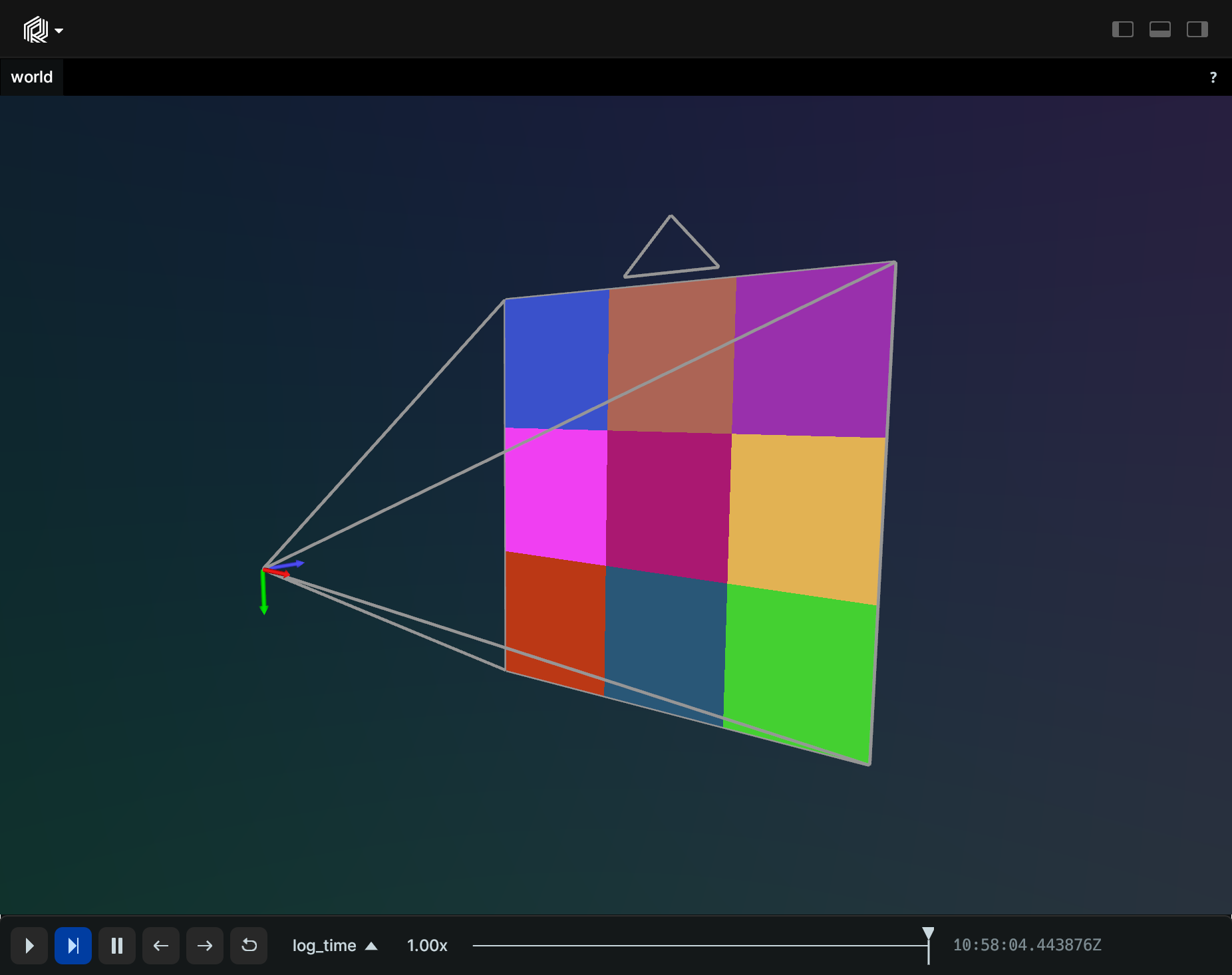# Pinhole

Camera perspective projection (a.k.a. intrinsics).

## Components

Required: `PinholeProjection`

Recommended: `Resolution`

Optional: `ViewCoordinates`

## Example

### Simple Pinhole Camera

``````"""Log a pinhole and a random image."""
import numpy as np
import rerun as rr

rr.init("rerun_example_pinhole", spawn=True)
rng = np.random.default_rng(12345)

image = rng.uniform(0, 255, size=[3, 3, 3])
rr.log("world/image", rr.Pinhole(focal_length=3, width=3, height=3))
rr.log("world/image", rr.Image(image))
``````